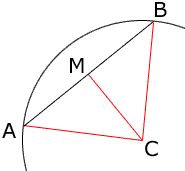Quandaries and Queries My question is based on geometry using recognizing arcs and chords. A chord of a circle is 48 centimeters long and is 10 centimeters from the center of the circle. Find the radius? I tried to refer back to examples in the book, but it did not help me. I hope to hear from you all again before tomorrow. Thanks a lot. Hi Aniesha, When you say the chord is "10 centimeters from the center of the circle" that this is the shortest distance from the center C of the circle to a point M on the chord. If this is so then the line CM is perpendicular to the chord.You can now show that the triangles CAM and CBM are congruent and hence the lengths of BM and MA are equal, and thus each 24 centimeters. Finally an application of Pythagoras' Theorem gives the length od CB which is a radius of the circle. Penny Go to Math Central